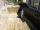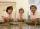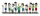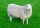# Fraction calculator

The calculator performs basic and advanced operations with fractions, expressions with fractions combined with integers, decimals, and mixed numbers. It also shows detailed step-by-step information about the fraction calculation procedure. Solve problems with two, three, or more fractions and numbers in one expression.

## Result:

### 6/7 - 2/3 = 4/21 ≅ 0.1904762

Spelled result in words is four twenty-firsts.

### How do you solve fractions step by step?

1. Subtract: 6/7 - 2/3 = 6 · 3/7 · 3 - 2 · 7/3 · 7 = 18/21 - 14/21 = 18 - 14/21 = 4/21
For adding, subtracting, and comparing fractions, it is suitable to adjust both fractions to a common (equal, identical) denominator. The common denominator you can calculate as the least common multiple of both denominators - LCM(7, 3) = 21. In practice, it is enough to find the common denominator (not necessarily the lowest) by multiplying the denominators: 7 × 3 = 21. In the next intermediate step, the fraction result cannot be further simplified by canceling.
In words - six sevenths minus two thirds = four twenty-firsts.

#### Rules for expressions with fractions:

Fractions - use the slash “/” between the numerator and denominator, i.e., for five-hundredths, enter 5/100. If you are using mixed numbers, be sure to leave a single space between the whole and fraction part.
The slash separates the numerator (number above a fraction line) and denominator (number below).

Mixed numerals (mixed fractions or mixed numbers) write as non-zero integer separated by one space and fraction i.e., 1 2/3 (having the same sign). An example of a negative mixed fraction: -5 1/2.
Because slash is both signs for fraction line and division, we recommended use colon (:) as the operator of division fractions i.e., 1/2 : 3.

Decimals (decimal numbers) enter with a decimal point . and they are automatically converted to fractions - i.e. 1.45.

The colon : and slash / is the symbol of division. Can be used to divide mixed numbers 1 2/3 : 4 3/8 or can be used for write complex fractions i.e. 1/2 : 1/3.
An asterisk * or × is the symbol for multiplication.
Plus + is addition, minus sign - is subtraction and ()[] is mathematical parentheses.
The exponentiation/power symbol is ^ - for example: (7/8-4/5)^2 = (7/8-4/5)2

#### Examples:

subtracting fractions: 2/3 - 1/2
multiplying fractions: 7/8 * 3/9
dividing Fractions: 1/2 : 3/4
exponentiation of fraction: 3/5^3
fractional exponents: 16 ^ 1/2
adding fractions and mixed numbers: 8/5 + 6 2/7
dividing integer and fraction: 5 ÷ 1/2
complex fractions: 5/8 : 2 2/3
decimal to fraction: 0.625
Fraction to Decimal: 1/4
Fraction to Percent: 1/8 %
comparing fractions: 1/4 2/3
multiplying a fraction by a whole number: 6 * 3/4
square root of a fraction: sqrt(1/16)
reducing or simplifying the fraction (simplification) - dividing the numerator and denominator of a fraction by the same non-zero number - equivalent fraction: 4/22
expression with brackets: 1/3 * (1/2 - 3 3/8)
compound fraction: 3/4 of 5/7
fractions multiple: 2/3 of 3/5
divide to find the quotient: 3/5 ÷ 2/3

The calculator follows well-known rules for order of operations. The most common mnemonics for remembering this order of operations are:
PEMDAS - Parentheses, Exponents, Multiplication, Division, Addition, Subtraction.
BEDMAS - Brackets, Exponents, Division, Multiplication, Addition, Subtraction
BODMAS - Brackets, Of or Order, Division, Multiplication, Addition, Subtraction.
GEMDAS - Grouping Symbols - brackets (){}, Exponents, Multiplication, Division, Addition, Subtraction.
Be careful, always do multiplication and division before addition and subtraction. Some operators (+ and -) and (* and /) has the same priority and then must evaluate from left to right.

## Fractions in word problems:

• FruitsAmy bought a basket of fruits 1/5 of them were apples,1/4 were oranges, and the rest were 33 bananas. How many fruits did she buy in all?
• DividendsThe three friends divided the win by the invested money. Karlos got three-eighths, John 320 permille, and the rest got Martin. Who got the most and which the least?
• Obtuse angleWhich obtuse angle is creating clocks at 17:00?
• ExpressionsLet k represent an unknown number, express the following expressions: 1. The sum of the number n and two 2. The quotient of the numbers n and nine 3. Twice the number n 4. The difference between nine and the number n 5. Nine less than the number n
• ExcavationExcavation for the base of the cottage has dimensions 4.5 m x 3.24 m x 60 cm. The excavated soil will increase its volume by one-quarter. Calculate the volume of excavated soil.
• DiscountThe dress was discounted by CZK 115, which was 12% of the original price. What is the cost of the dress after the discount?
• University bubbleYou'll notice that the college up slowly every other high school. In Slovakia/Czech republic a lot of people studying political science, mass media communication, social work, many sorts of management MBA. Calculate how many times more earns clever 25-yea
• The thirdThe one-third rod is blue, one-half of the rod is red, the rest of the rod is white and measures 8 cm. How long is the whole rod?
• ExpressionIf it is true that (l + 15 w)/(w) = 6, then the value of the expression (13 l)/(12 w) is:
• CooksFour cooks cleaned 5 kg of potatoes for 10 minutes. How many cook would have to work clean 9 kg of potatoes for 12 minutes?
• Children 9There are 11 children in a room. 6 of the children are girls. What fraction of the children are girls?
• There 13There are 7 cows 3 sheeps and 4 chickens on a field . What fraction of the animals are sheep?
• Water 31Richard takes 3 1/6 liters of water before noon and 2 3/5 liters of water afternoon. How many liters of water does Richard consume a day?